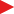## Please enable JavaScript to view this site.

math channel

Note: If you need output values in imperial units the equation will need to include a value conversion.

name

Name of the math channels.

unit

The unit to be used after the final value, can be anything. Will be visible in the logger.

resolution

Specifies the sensor resolution on the output value.

1 (-32768 to +32767) - Values between -32768 and + 32767 can be used on the table (no decimals).

0.1 (-3276.8 to +3276.7) - Values between -3276.8 and + 3276.7 can be used on the table (one decimal).

0.01 (-327.68 to +327.67) - Values between -327.68 and + 327.67 can be used on the table (two decimals).

0.001 (-32.768 to +32.767) - Values between -32.768 and + 32.767 can be used on the table (three decimals).

Note: The resolution is on the output value only, not the input values.

Warning/Note: When using a MaxxECU RealTime Data value in any in or output function, including comparing, the resolution (scaling) on all the values involved needs to use the same resolution values.How to find the resolutionHow the ECU uses the resolutionExample of bad practice

In short, always try to use the same resolution all over the sensors and tables. 0.1 is the most used resolution used across whole MaxxECU.

Exponential smoothing alpha

Alpha smoothing value as an extra filtering option. A to high value will slow down the response.

0.0% = no smoothing

90. - 95.0% = recommended smoothing value.Smoothing examples

Enable

The math formula to be used on this channel.

term x

term type

Use a constant value or an RealTime Data value.

constant - Uses a constant value in the math channel.

Variable - Uses as a RealTime Data value in the math channel.

constant X

The constant value to be used.

variable X

The variable to be used.